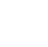Back to Chapter
Getting StartedBasic Syntax & DeclarationControl StatementsNo items found.
Function & EventsNo items found.
Array and its TypesNo items found.
OOP : Object Oriented ProgrammingNo items found.
Javascript StandardsNo items found.
HTML DOMNo items found.No items found.No items found.No items found.

## Prompt

Let me introduce you to the new Object in javascript i.e. prompt(" ")

It is Used to Take input from the user, for example you want to take the name as input and print the  Hello personName, to do this you have to use prompt

Syntax

``` var variableName = prompt("String to Ask"); ```

Example:

``` var name = prompt("Please Enter Your Name."); console.log(name); ```

Explanation :

First We have declared a new variable name and have used prompt function to take input from the user.

Then we are printing that name in the console tab.

If you are taking Numbers as the input in variable, then to consider it as a number you have to use parseInt function to convert var to Number

Example (Incorrect)

``` var num1 = prompt("Enter 1st Number") var num2 = prompt("Enter 2nd Number") var total = num1 + num2; console.log(total); //Input // 5 // 10 //Output // 510 ```

Example (Correct)

``` var num1 = prompt("Enter 1st Number") var num2 = prompt("Enter 2nd Number") var total = parseInt(num1) + parseInt(num2); console.log(total); //Input // 5 // 10 //Output // 15 ```

Here,

i have use parseInt function so input number will be considered as a number.